## The Ultimate Guide to Math Fact Fluency

Students counting on their fingers is a sure-tell sign that they didn’t acquire math fact fluency. It is sad to see students, ashamed of the only thing they know, counting on their fingers under their desks. Our elementary educational mission is failing students who haven’t developed math fact fluency, which is the foundation to more advanced math skills.

Developing math fact fluency takes structure, organization, and work on the part of both teachers and students. In this article, I will share everything you need to know about developing math fact fluency.

## What is Math Fact Fluency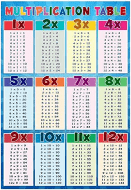Math facts are single-digit problems such as 7+9 or 6×8 or 14-5, and so on. A common name for all the multiplication math facts is the “multiplication table.” Math fact fluency is the ability to answer all math fact questions instantly from recall without having to think through the problem.

Students should be able to recall math facts instantly without having to count on their fingers or hesitate to think about the answer. This may seem like a high bar, but our brains are great at recalling an unbelievable amount of information daily, and with practice, math facts can be recalled the same way.

## Three Reasons Why Math Fact Fluency is Important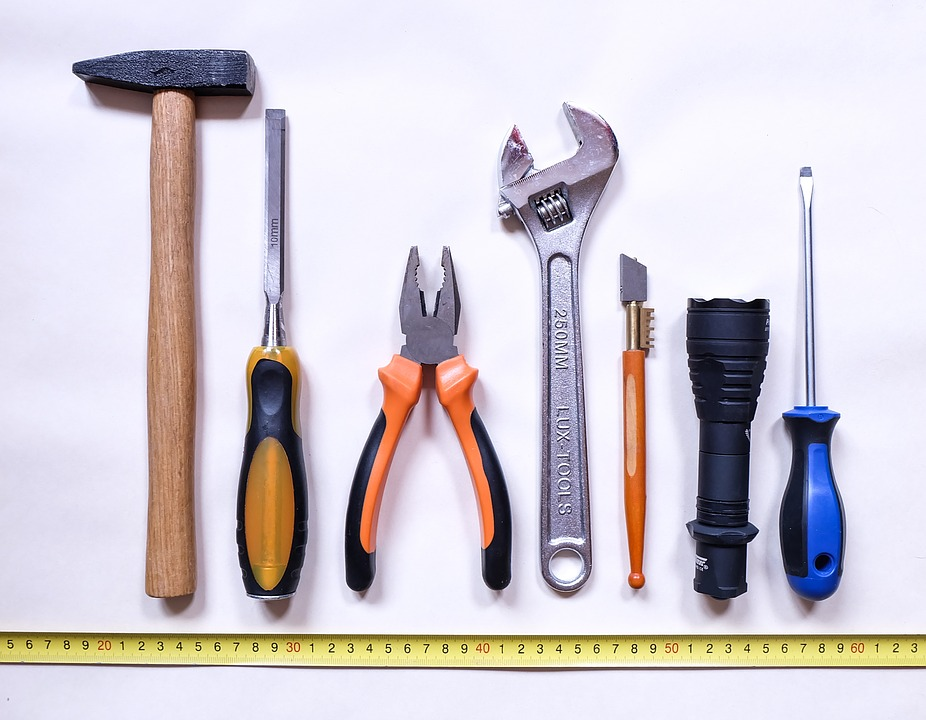Math fact fluency is critical because it is a “tool skill.” Meaning it is a tool used in the process of doing other math problems. Developing this tool skill makes learning math easier as concepts get more complicated. This tool skill needs to be automatic in the student’s brain to save precious short-term memory resources.

Math fact fluency can be compared to reading. Students must recognize words automatically to comprehend the author’s meaning. Otherwise, they will spend too much time decoding individual words.

When students are fluent in math facts, they are focused on the math process as a whole rather than stopping to puzzle out the facts. This is important for three reasons:

### 1. Students with math fact fluency make fewer errors

Students who lack math fact fluency often make careless errors doing arithmetic computations. If they devote too much energy to deriving math facts, they lose sight of the problem at hand and make mistakes that would otherwise be obvious. Those who can effortlessly recall math facts can concentrate on what they are doing and ultimately make fewer errors.

### 2. Math fact fluency makes learning math easier

When a new math procedure is introduced, students who have math fact fluency can easily follow the thread of instruction. Without this fluency, students fall behind instruction or demonstrations as they try working out math facts. This distraction takes away from a student absorbing all of the details necessary to successfully learn new math processes.

The first teacher to use Rocket Math to teach subtraction facts to her second graders realized the benefit first hand. She told me that with Rocket Math, she was able to teach regrouping in subtraction in just three days.

Her students mastered the math facts, and the outcome was extraordinary. The teacher shared that since these students had developed fluency in subtraction facts, they were able to learn other procedures easily.

### 3. Students who have developed math fact fluency enjoy math and always complete their work

Having to count on your fingers or look up facts on a timetable is slow and onerous. When students can’t work quickly, math problems become a dreaded drudgery. Students are motivated by mastering new skills, which will help them work faster and build confidence. Those who can quickly recall math facts will complete their work with ease and enjoy the feeling of accomplishment.

## How to Build and Improve Math Fact FluencyBuilding and improving math fact fluency requires a systematic effort over the elementary years. It is a long climb to achieve mastery and there are no short-cuts.

Consistent daily practice throughout elementary school is important for retention. Slow and steady wins the race when building math fact fluency.

Math fact practice should be structured in such a way that students are learning a small number of facts at a time. These small groups of facts should be practiced daily until students have reached mastery. As time goes on, more groups of math facts are introduced systematically in small amounts for students to master.

Learning the 0 through 9s facts in the four basic operations will take elementary students months to master. Worksheets and game applications are two of the best ways to teach fact fluency over time. Combining structured math fact learning, practice, and evaluation with fun math fact games helps students develop number sense and understand complex numerical relationships.

## Teaching Math Fact Fluency with WorksheetsWorksheets are popular tools that teachers reach for when teaching math facts, but sadly, they often fall short for the majority of students. A few select students will begin memorizing the facts on their own accord in order to make the worksheets easier, but most students will continue to slowly work out the facts either on their fingers or in their heads. These students may never develop a strong recall of the facts and become flustered when asked to answer problems on the spot.

Fortunately, there are specific worksheets that are effective in building fact fluency. The key is having worksheets that are structured, systematic, and sequenced. Each worksheet should only have two to four facts to be learned.

By working on only two to four facts, these worksheets help teach memorization for a strong recall, rather than reinforcing working out problems slowly. Students will then be able to remember these small groups of facts easier, and by the end of the worksheet will be writing answers from memory.

## Teaching Math Fact Fluency with The Rocket Math Worksheet ProgramThe Rocket Math Worksheet Program improves upon this concept by using paired practice and saying facts aloud. Students partner up and practice quickly recalling facts together. One student asks the questions and watches for when their partner hesitates to answer. He or she then gives his or her partner more opportunities to practice any fact that isn’t coming to mind instantly.

The students switch roles, and after both have answered questions, they then take a one minute test on the facts that they have learned so far. If students are answering as fast as their fingers will carry them, then they pass the level and move on to the next worksheet in the sequence.

Ten minutes of practice every day gets the job done, especially when paired with using these facts in higher level math problems.

## Teaching Math Fact Fluency with Games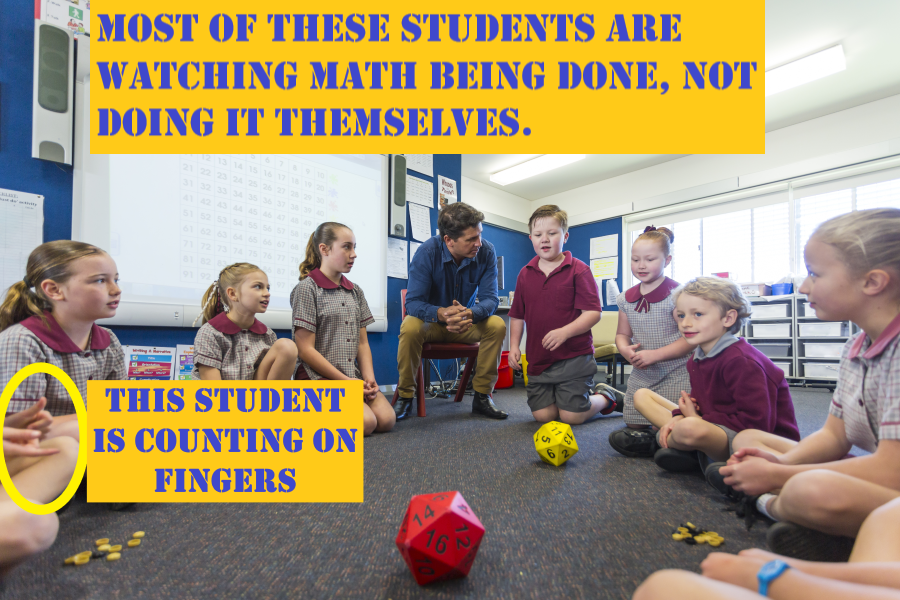In addition to worksheets, schools of education tell teachers to use games to “teach” math facts. Unfortunately, most games and fun activities do not actually help individual students learning math facts to the level of fluency. These games, such as bingo or dice, have several fallouts:

• Students spend most of their time waiting for their turn rather than practicing facts.
• The games do not focus on teaching a small group of facts in a manner that helps students commit them to memory.
• The games do not adjust to an individual student’s level of fluency.
• Students can pace the game slowly enough to have time to figure out facts rather than requiring recall.
• It is difficult to keep every student engaged, as those who are behind are less likely to participate.

## Using the Rocket Math Online Game as an Effective Way to Teach Math Fact Fluency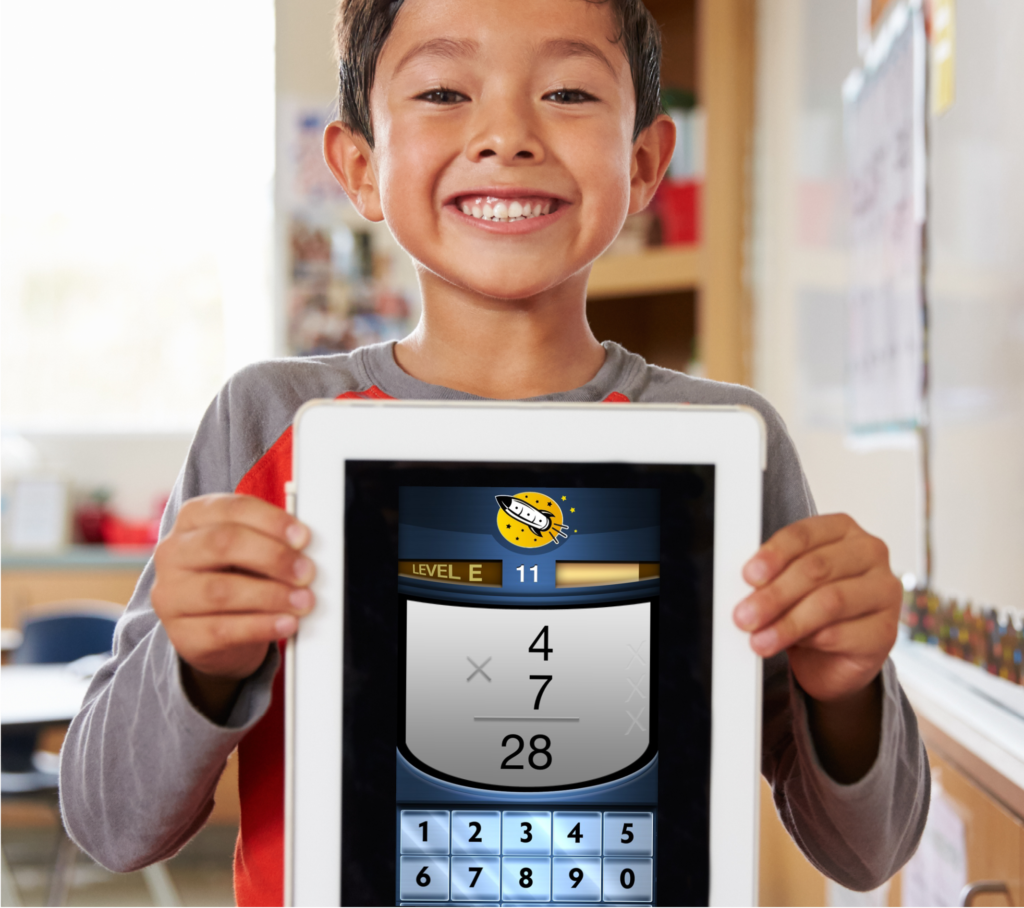There are games that are very effective at building math fact fluency. Games such as the Rocket Math Online Game have several important features that make a big difference.

1. Every student is engaged in answering math facts—not waiting for a turn.
2. Students learn only a few new facts at a time so that they can remember them.
3. The game provides lots of focused practice on each set of facts.
4. The game requires students to answer quickly, which guarantees the students recall the answer rather than “figuring it out” over and over.
5. The game gives an immediate correction and extra practice on any facts that students cannot answer quickly and correctly.
6. The game only introduces new facts once students demonstrate mastery of facts learned so far.
7. The game gives students explicit feedback so they have a sense of accomplishment as they work their way through an operation.

## Math Fact Fluency Benchmarks

The following benchmarks are reasonable expectations for a school that has an effective math fluency program in place. Of course, a student cannot write math facts any faster than they can normally write, so take that into account when looking at fluency benchmarks. Adjust the benchmarks for students who do not write quickly.

### Kindergarten Numeral Writing Fluency Benchmarks (digits)

Start of Year Mid-Year End of Year
20 digits per minute 40 digits per minute

### First Grade Numeral Writing Fluency Benchmarks (digits)

Start of Year Mid-Year End of Year
40 digits per minute 60 digits per minute 60 digits per minute

### First Grade Math Fact Fluency Benchmarks (problems per minute)

Start of Year Mid-Year End of Year

### Second Grade Math Fact Fluency (problems per minute)

Start of Year Mid-Year End of Year
Subtraction: 12 per minute Subtraction: 25 per minute

### Third Grade Math Fact Fluency Benchmarks (problems per minute)

Start of Year Mid-Year End of Year
Subtraction: 30 per minute Subtraction: 30 per minute Subtraction: 30 per minute
Multiplication: 30 per minute Multiplication: 30 per minute

### Fourth Grade Math Fact Fluency Benchmarks (problems per minute)

Start of Year Mid-Year End of Year
Subtraction: 35 per minute Subtraction: 35 per minute Subtraction: 35 per minute
Multiplication: 35 per minute Multiplication: 35 per minute Multiplication: 35 per minute
Division: 20 per minute Division: 35 per minute

### Fifth Grade Math Fact Fluency Benchmarks (problems per minute)

Start of Year Mid-Year End of Year
Subtraction: 35 per minute Subtraction: 40 per minute Subtraction: 40 per minute
Multiplication: 35 per minute Multiplication: 40 per minute Multiplication: 40 per minute
Division: 35 per minute Division: 40 per minute Division: 40 per minute

Math Fact Fluency Assessment

Use this printable packet of free math fact fluency assessments to test your students’ skill levels relative to  the above benchmarks. This will give you a clear idea of your students’ fluency and where there is room for opportunity.

Rocket Math’s assessment packet includes a writing speed test, which helps create realistic expectations for individual students. Using the goal sheet ensures you will evaluate the individual student math fact fluency in light of their writing speed.

Rate students as:

1. Weak, needs fact work
2. Good, but fact work could help
3. Strong, fact work not needed

Special triage priority: if you have fourth-grade students and above, start with multiplication facts. Multiplication facts are essential to future success in math above fourth grade. Even if fourth graders are counting on their fingers for addition and subtraction, teach multiplication mastery first. If fourth graders move to the next grade without strong multiplication fact fluency, they will have a hard time successfully progressing through math.

## The Best Tools for Developing Math Fact Fluency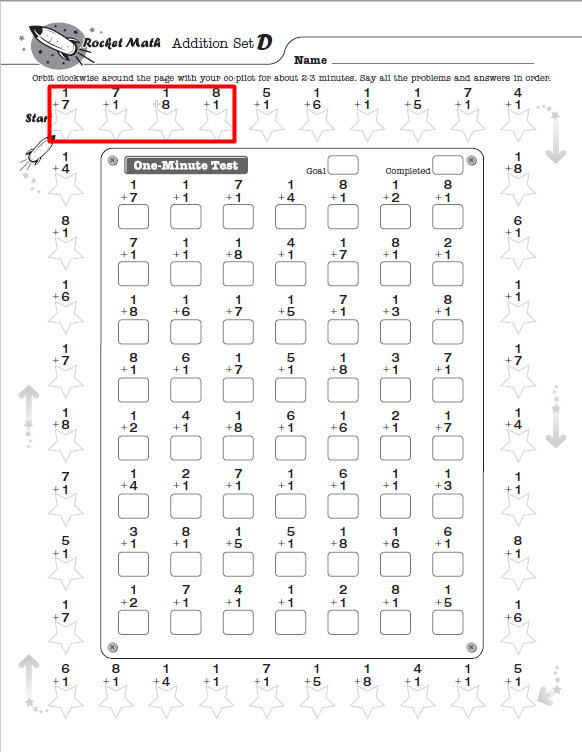With the right tools, any student can develop math fact fluency and have fun while doing it! Students use Rocket Math’s Subscription Worksheet Program to practice with partners, then take timed tests. Rocket Math also offers math facts practice online through the Rocket Math Online Game. Students can log in and play from any device, anywhere, any time of day! Start a free trial today.

Both the worksheet program and the online game help students master addition, subtraction, multiplication, and division math facts for a lifetime of success in math.